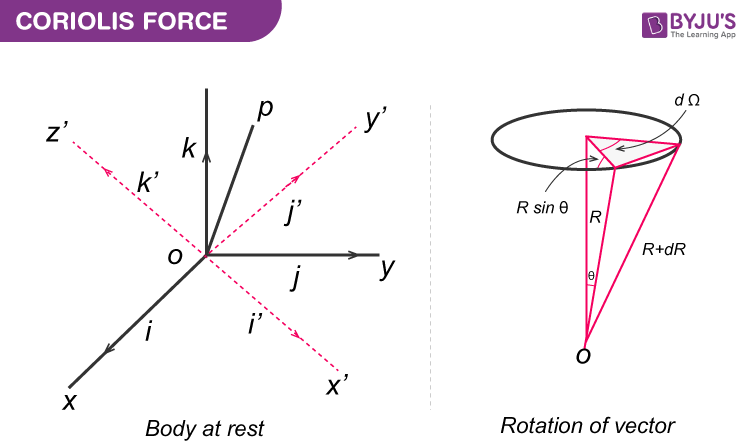Checkout JEE MAINS 2022 Question Paper Analysis : Checkout JEE MAINS 2022 Question Paper Analysis :

# Coriolis Force Derivation

Coriolis force is an inertial or fictitious force that acts on the object with velocity

$$\begin{array}{l}\vec{V}\end{array}$$
that are in motion within the frame of reference. If the frame of reference has a clockwise rotation, then the force acts to the left of the object and if the frame of reference has an anti-clockwise rotation, the force acts to the right of the object.

## Derivation of Coriolis Force

Consider two stationary frames of reference, one in space as x,y,z and the other in the body as x’,y’,z’. At time t=0, both the reference frames coincide.The position vector at any given point P (x,y,z) in the stationary coordinate system is given as

r=r(t)

Where,

For space set of axes:

$$\begin{array}{l}r=x\hat{i}+y\hat{j}+z\hat{k}\end{array}$$

For body set of axes:

$$\begin{array}{l}r={x}’\hat{i}+{y}’\hat{j}+{z}’\hat{k}\end{array}$$

For O(x’,y’,z’) system there is a change in i’,j’,k’ with a change in time but i,j,k are stationary.

The angular velocity

$$\begin{array}{l}\vec{\omega }\end{array}$$
is obtained as:

$$\begin{array}{l}dR=Rsin\Theta d\Omega\end{array}$$
$$\begin{array}{l}dR=d\vec{\Omega }*R\end{array}$$
$$\begin{array}{l}\frac{dR}{dt}=\frac{d\vec{\Omega }}{dt}*R\end{array}$$

=

$$\begin{array}{l}\vec{\omega }*R\end{array}$$
$$\begin{array}{l}\frac{d{i}’}{dt}=\vec{\omega }*{i}'\end{array}$$

When the stationary system rotates with the angular velocity ꞷ, the rate of change of vector r with respect to system in motion is given:

$$\begin{array}{l}\frac{dr}{dt}=\frac{d}{dt}({i}'{x}’+{j}'{y}’+{k}'{z}’)\end{array}$$

=

$$\begin{array}{l}{x}'{i}’+{y}'{j}’+{z}'{k}’+{x}’\frac{d{i}’}{dt}+{y}’\frac{d{j}’}{dt}+{z}’\frac{d{k}’}{dt}\end{array}$$

=

$$\begin{array}{l}{x}'{i}’+{y}'{j}’+{z}'{k}’+{x}'(\vec{\omega *{i}’})+{y}'(\vec{\omega *{j}’})+{z}'(\vec{\omega *{k}’})\end{array}$$

=

$$\begin{array}{l}{x}'{i}’+{y}'{j}’+{z}'{k}’+\vec{\omega }*r\end{array}$$
$$\begin{array}{l}(\frac{dr}{dt})_{motion}=(\frac{dr}{dt})_{stationary}+\vec{\omega }*r\end{array}$$
$$\begin{array}{l}(\frac{dr}{dt})=(\frac{{d}’r}{dt})+\vec{\omega }*r\end{array}$$
(eq.1)

Equation 1. is obtained by applying time derivative to the vector velocity. After differentiating the equation on both sides with respect to time we get,

$$\begin{array}{l}\frac{d}{dr}(\frac{dr}{dt})=(\frac{{d^{2}}’r}{dt^{2}})=\frac{d}{dt}(\frac{{d}’r}{dt}+\vec{\omega }*r)\end{array}$$
$$\begin{array}{l}\frac{{d}’^{2}r}{dt^{2}}+(\frac{{d}’\omega }{dt}*r+\vec{\omega }\frac{{d}’r}{dt})+(\vec{\omega }\frac{{d}’r}{dt})+\vec{\omega }(\vec{\omega }*r)\end{array}$$
$$\begin{array}{l}\frac{{d}’^{2}r}{dt^{2}}+2\vec{\omega }\frac{{d}’r}{dt}+\vec{\omega }(\vec{\omega }*r)+\frac{{d}’\vec{\omega }}{dt}r\end{array}$$
(eq.2)

In equation 2.

$$\begin{array}{l}\vec{\omega }*(\vec{\omega }*r)\end{array}$$
is the centripetal acceleration which is given as:

$$\begin{array}{l}\left | \vec{\omega }*(\vec{\omega }*r) \right |=\omega ^{2}rsin\Theta =\frac{v^{2}}{rsin\Theta }\end{array}$$

The second term is known as Coriolis acceleration which is present when point r is in motion.

Therefore, Coriolis acceleration is given as:

$$\begin{array}{l}2\vec{\omega }*\frac{{d}’r}{dt}\end{array}$$

From Newton’s laws of motion for moving system we have,

F=ma

$$\begin{array}{l}F=m\frac{d^{2}r}{dt^{2}}+m\vec{\omega }(\vec{\omega }r)+2m\vec{\omega }(\frac{{d}’r}{dt})+m\frac{d\vec{\omega }}{dt}r\end{array}$$

From Newton’s laws of motion for stationary system we have,

$$\begin{array}{l}m\frac{d^{2}r}{dt^{2}}=F-[m\vec{\omega }(\vec{\omega }r)+2m\vec{\omega }\frac{{d}’r}{dt}+m\frac{d\vec{\omega }}{dt}r]\end{array}$$
(eq.3)

By substituting for:

$$\begin{array}{l}m\frac{d^{2}r}{dt^{2}}=F_{eff}\end{array}$$
$$\begin{array}{l}\frac{{d}’r}{dt}={v}'\end{array}$$
$$\begin{array}{l}F_{eff}=F-2m(\vec{\omega }*{v}’)-m(\vec{\omega }(\vec{\omega} *r))-m\frac{d\vec{\omega }}{dt}r\end{array}$$

The second term

$$\begin{array}{l}2m(\vec{\omega }*{v}’)\end{array}$$
is known as the Coriolis force.

## Coriolis Effect and its Causes

The deflection of an object due to the Coriolis force is called the Coriolis effect. The Coriolis effect is used to describe the Coriolis force experienced by the moving objects such that the force is acting perpendicular to the direction of motion and to the axis of rotation. The earth’s rotation is the main cause for the Coriolis effect as the earth rotates faster at the equator and near the poles the rotation is sluggish. This is because the rate of change in the diameter of the earth’s latitude increases near the poles.

The air currents in the Northern hemisphere bend to the right making the objects deflect to the right whereas in the Southern hemisphere the air currents bend to the left making the objects deflect to the left. The Coriolis effect is noticed only for the motions occurring at large-scale such as movement of air and water in the ocean. Example of Coriolis effect is change in weather patterns.

Stay tuned with BYJU’S to learn more on other Physics related concepts.

Related Physics Articles:

## Frequently Asked Questions – FAQs

### State Newton’s second law of motion?

Newton’s second law of motion states “Force is equal to the rate of change of momentum. For a constant mass, force equals mass times acceleration”.

### What is coriolis force?

The force experienced by the objects moving in fixed rotating coordinates is known as coriolis force.

### Give an example for coriolis force

Change in whether pattern is an example for coriolis force.

Yes.

### State true or false: The Coriolis effect is noticed in the movement of air and water in the ocean.

True.

Test Your Knowledge On Coriolis Force Derivation!